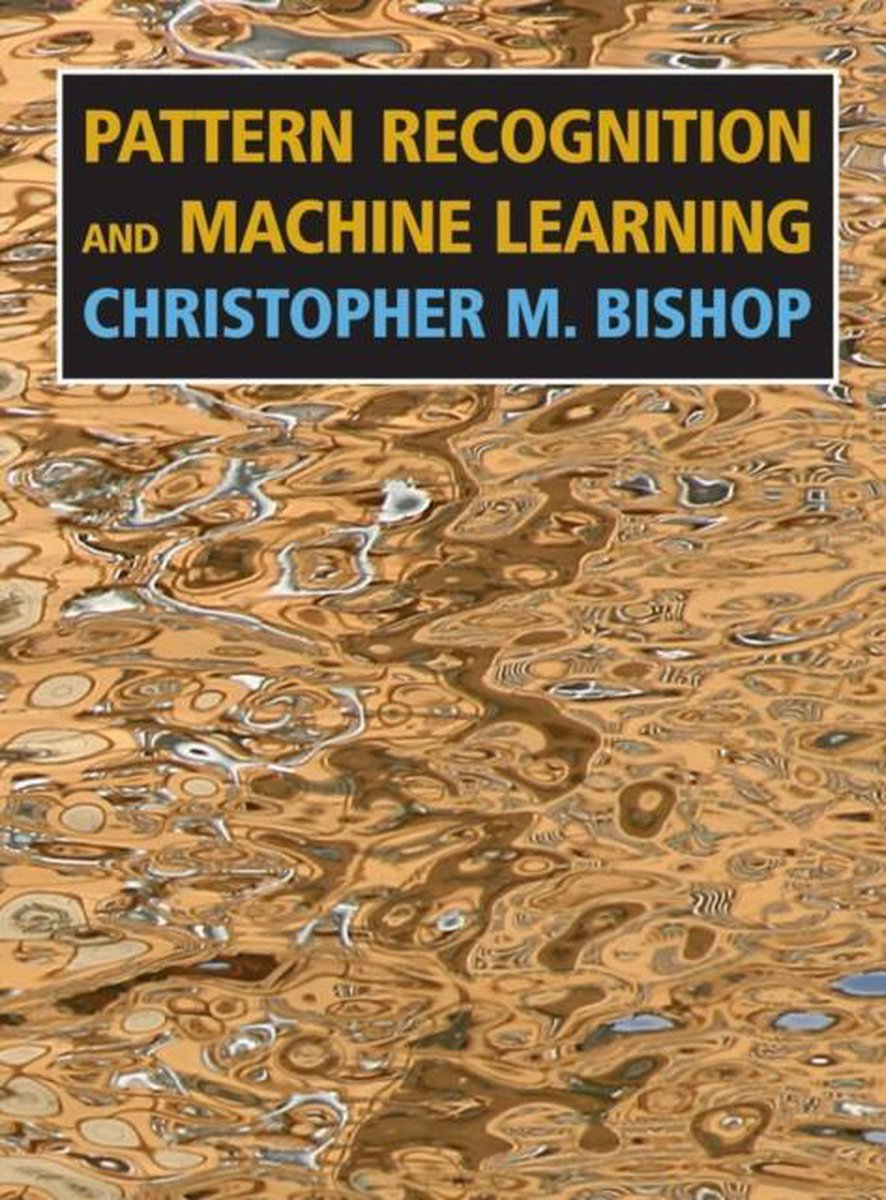•Pattern Recognition and Machine Learning

Christopher M. Bishop

This is the first textbook on pattern recognition to present the Bayesian viewpoint. The book presents approximate inference algorithms that permit fast approximate answers in situations where exact answers are not feasible. It uses graphical models to describe probability distributions when no other books apply graphical models to machine learning. No previous knowledge of pattern recognition or machine learning concepts is assumed. Familiarity with multivariate calculus and basic linear algebra is required, and some experience in the use of probabilities would be helpful though not essential as the book includes a self-contained introduction to basic probability theory.

• Ean/ISBN: 9780387310732
• Review: